### Create an Account

Home / Questions / Electric Power and Sign Convention 218 In the circuits of Figure P218 the directions...

# Electric Power and Sign Convention 218 In the circuits of Figure P218 the directions of current and polarities of voltage have already been defined Find the actual values of the indicated

#### Electric Power and Sign Convention

2.18      In the circuits of Figure P2.18, the directions of current and polarities of voltage have already been defined. Find the actual values of the indicated currents and voltages.

2.19      Find the power delivered by each source in the circuits of Figure P2.19.

V(t) = 12 – 3e–5t/12 hrs

i              6 A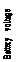12 V5 AV

2 A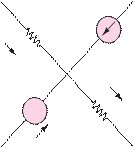12 h                t

Figure P2.15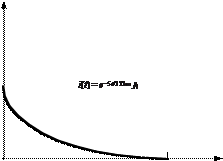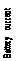+     3 V                                  10 V    +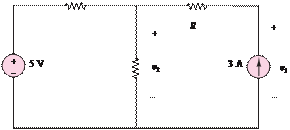1 A

12 h                t

Figure P2.12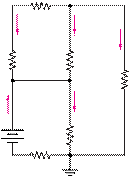R4             a

Figure P2.16

I0                          I1

I2

R0                          R1

 2

R

b

I3

IS

+

VS                                                            R3

-

R5

10  A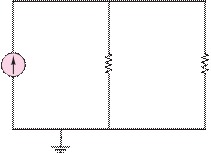30 Ù15 Ù               I1

Figure P2.13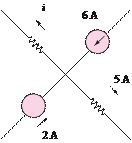Figure P2.17

Jun 19 2020 View more View LessSubscribe To Get Solution